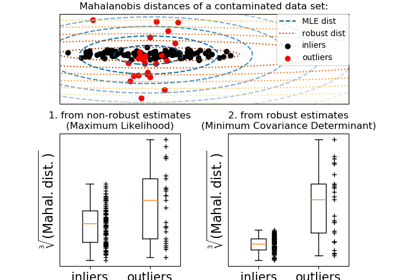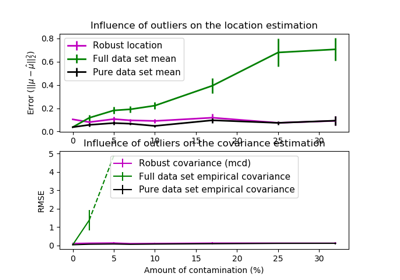# sklearn.covariance.MinCovDet¶

class sklearn.covariance.MinCovDet(store_precision=True, assume_centered=False, support_fraction=None, random_state=None)[source]

Minimum Covariance Determinant (MCD): robust estimator of covariance.

The Minimum Covariance Determinant covariance estimator is to be applied on Gaussian-distributed data, but could still be relevant on data drawn from a unimodal, symmetric distribution. It is not meant to be used with multi-modal data (the algorithm used to fit a MinCovDet object is likely to fail in such a case). One should consider projection pursuit methods to deal with multi-modal datasets.

Read more in the User Guide.

Parameters: store_precision : bool Specify if the estimated precision is stored. assume_centered : bool If True, the support of the robust location and the covariance estimates is computed, and a covariance estimate is recomputed from it, without centering the data. Useful to work with data whose mean is significantly equal to zero but is not exactly zero. If False, the robust location and covariance are directly computed with the FastMCD algorithm without additional treatment. support_fraction : float, 0 < support_fraction < 1 The proportion of points to be included in the support of the raw MCD estimate. Default is None, which implies that the minimum value of support_fraction will be used within the algorithm: [n_sample + n_features + 1] / 2 random_state : int, RandomState instance or None, optional (default=None) If int, random_state is the seed used by the random number generator; If RandomState instance, random_state is the random number generator; If None, the random number generator is the RandomState instance used by np.random. raw_location_ : array-like, shape (n_features,) The raw robust estimated location before correction and re-weighting. raw_covariance_ : array-like, shape (n_features, n_features) The raw robust estimated covariance before correction and re-weighting. raw_support_ : array-like, shape (n_samples,) A mask of the observations that have been used to compute the raw robust estimates of location and shape, before correction and re-weighting. location_ : array-like, shape (n_features,) Estimated robust location covariance_ : array-like, shape (n_features, n_features) Estimated robust covariance matrix precision_ : array-like, shape (n_features, n_features) Estimated pseudo inverse matrix. (stored only if store_precision is True) support_ : array-like, shape (n_samples,) A mask of the observations that have been used to compute the robust estimates of location and shape. dist_ : array-like, shape (n_samples,) Mahalanobis distances of the training set (on which fit is called) observations.

References

 [Rouseeuw1984] P. J. Rousseeuw. Least median of squares regression. J. Am Stat Ass, 79:871, 1984.
 [Rousseeuw] A Fast Algorithm for the Minimum Covariance Determinant Estimator, 1999, American Statistical Association and the American Society for Quality, TECHNOMETRICS
 [ButlerDavies] R. W. Butler, P. L. Davies and M. Jhun, Asymptotics For The Minimum Covariance Determinant Estimator, The Annals of Statistics, 1993, Vol. 21, No. 3, 1385-1400

Examples

>>> import numpy as np
>>> from sklearn.covariance import MinCovDet
>>> from sklearn.datasets import make_gaussian_quantiles
>>> real_cov = np.array([[.8, .3],
...                      [.3, .4]])
>>> np.random.seed(0)
>>> X = np.random.multivariate_normal(mean=[0, 0],
...                                   cov=real_cov,
...                                   size=500)
>>> cov = MinCovDet(random_state=0).fit(X)
>>> cov.covariance_
array([[0.7411..., 0.2535...],
[0.2535..., 0.3053...]])
>>> cov.location_
array([0.0813... , 0.0427...])


Methods

 correct_covariance(data) Apply a correction to raw Minimum Covariance Determinant estimates. error_norm(comp_cov[, norm, scaling, squared]) Computes the Mean Squared Error between two covariance estimators. fit(X[, y]) Fits a Minimum Covariance Determinant with the FastMCD algorithm. get_params([deep]) Get parameters for this estimator. get_precision() Getter for the precision matrix. mahalanobis(X) Computes the squared Mahalanobis distances of given observations. reweight_covariance(data) Re-weight raw Minimum Covariance Determinant estimates. score(X_test[, y]) Computes the log-likelihood of a Gaussian data set with self.covariance_ as an estimator of its covariance matrix. set_params(**params) Set the parameters of this estimator.
__init__(store_precision=True, assume_centered=False, support_fraction=None, random_state=None)[source]
correct_covariance(data)[source]

Apply a correction to raw Minimum Covariance Determinant estimates.

Correction using the empirical correction factor suggested by Rousseeuw and Van Driessen in [RVD].

Parameters: data : array-like, shape (n_samples, n_features) The data matrix, with p features and n samples. The data set must be the one which was used to compute the raw estimates. covariance_corrected : array-like, shape (n_features, n_features) Corrected robust covariance estimate.

References

 [RVD] (1, 2) A Fast Algorithm for the Minimum Covariance Determinant Estimator, 1999, American Statistical Association and the American Society for Quality, TECHNOMETRICS
error_norm(comp_cov, norm='frobenius', scaling=True, squared=True)[source]

Computes the Mean Squared Error between two covariance estimators. (In the sense of the Frobenius norm).

Parameters: comp_cov : array-like, shape = [n_features, n_features] The covariance to compare with. norm : str The type of norm used to compute the error. Available error types: - ‘frobenius’ (default): sqrt(tr(A^t.A)) - ‘spectral’: sqrt(max(eigenvalues(A^t.A)) where A is the error (comp_cov - self.covariance_). scaling : bool If True (default), the squared error norm is divided by n_features. If False, the squared error norm is not rescaled. squared : bool Whether to compute the squared error norm or the error norm. If True (default), the squared error norm is returned. If False, the error norm is returned. The Mean Squared Error (in the sense of the Frobenius norm) between self and comp_cov covariance estimators.
fit(X, y=None)[source]

Fits a Minimum Covariance Determinant with the FastMCD algorithm.

Parameters: X : array-like, shape = [n_samples, n_features] Training data, where n_samples is the number of samples and n_features is the number of features. y not used, present for API consistence purpose. self : object
get_params(deep=True)[source]

Get parameters for this estimator.

Parameters: deep : boolean, optional If True, will return the parameters for this estimator and contained subobjects that are estimators. params : mapping of string to any Parameter names mapped to their values.
get_precision()[source]

Getter for the precision matrix.

Returns: precision_ : array-like The precision matrix associated to the current covariance object.
mahalanobis(X)[source]

Computes the squared Mahalanobis distances of given observations.

Parameters: X : array-like, shape = [n_samples, n_features] The observations, the Mahalanobis distances of the which we compute. Observations are assumed to be drawn from the same distribution than the data used in fit. dist : array, shape = [n_samples,] Squared Mahalanobis distances of the observations.
reweight_covariance(data)[source]

Re-weight raw Minimum Covariance Determinant estimates.

Re-weight observations using Rousseeuw’s method (equivalent to deleting outlying observations from the data set before computing location and covariance estimates) described in [RVDriessen].

Parameters: data : array-like, shape (n_samples, n_features) The data matrix, with p features and n samples. The data set must be the one which was used to compute the raw estimates. location_reweighted : array-like, shape (n_features, ) Re-weighted robust location estimate. covariance_reweighted : array-like, shape (n_features, n_features) Re-weighted robust covariance estimate. support_reweighted : array-like, type boolean, shape (n_samples,) A mask of the observations that have been used to compute the re-weighted robust location and covariance estimates.

References

 [RVDriessen] (1, 2) A Fast Algorithm for the Minimum Covariance Determinant Estimator, 1999, American Statistical Association and the American Society for Quality, TECHNOMETRICS
score(X_test, y=None)[source]

Computes the log-likelihood of a Gaussian data set with self.covariance_ as an estimator of its covariance matrix.

Parameters: X_test : array-like, shape = [n_samples, n_features] Test data of which we compute the likelihood, where n_samples is the number of samples and n_features is the number of features. X_test is assumed to be drawn from the same distribution than the data used in fit (including centering). y not used, present for API consistence purpose. res : float The likelihood of the data set with self.covariance_ as an estimator of its covariance matrix.
set_params(**params)[source]

Set the parameters of this estimator.

The method works on simple estimators as well as on nested objects (such as pipelines). The latter have parameters of the form <component>__<parameter> so that it’s possible to update each component of a nested object.

Returns: self

## Examples using sklearn.covariance.MinCovDet¶Robust covariance estimation and Mahalanobis distances relevanceRobust vs Empirical covariance estimate